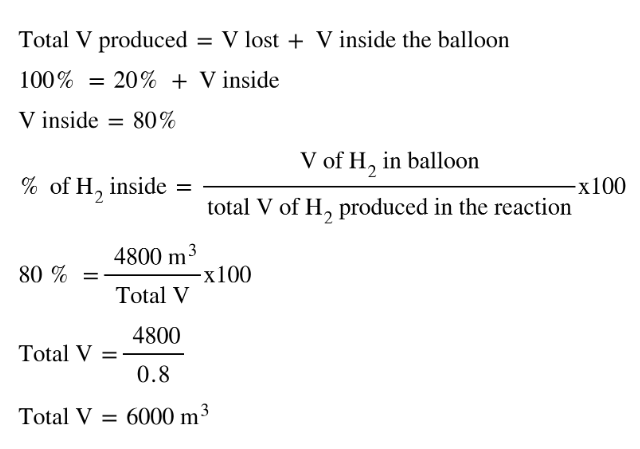# Problem: In 1897 the Swedish explorer Andreé tried to reach the North Pole in a balloon. The balloon was filled with hydrogen gas. The hydrogen gas was prepared from iron splints and diluted sulfuric acid. The reaction isFe(s) + H2SO4(aq) → FeSO4(aq) + H2(g)The volume of the balloon was 4800 m3 and the loss of hydrogen gas during filling was estimated at 20.%. What mass of iron splints and 98% (by mass) H2SO4 were needed to ensure the complete filling of the balloon? Assume a temperature of 0°C, a pressure of 1.0 atm during filling, and 100% yield.

###### FREE Expert Solution
• First need find how much moles of H2 does a 4800 m3 balloon contain.
• We will be using the ideal gas equation to find the moles of H2 using the conditions provided
• The moles of H2 is related to the moles of Fe and H2SO4 using the balanced equation provided which in turn will give us the mass Fe and H2SO
• For the volume of H2, if there was 20% loss, this means that 4800 m3 is 80% of the total H2 that was produced (assuming that 20% is in volume as well)99% (116 ratings)###### Problem Details

In 1897 the Swedish explorer Andreé tried to reach the North Pole in a balloon. The balloon was filled with hydrogen gas. The hydrogen gas was prepared from iron splints and diluted sulfuric acid. The reaction is

Fe(s) + H2SO4(aq) → FeSO4(aq) + H2(g)

The volume of the balloon was 4800 m3 and the loss of hydrogen gas during filling was estimated at 20.%. What mass of iron splints and 98% (by mass) H2SO4 were needed to ensure the complete filling of the balloon? Assume a temperature of 0°C, a pressure of 1.0 atm during filling, and 100% yield.